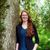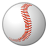# QlikView App Dev

Discussion Board for collaboration related to QlikView App Development.

cancel
Showing results for
Did you mean:Not applicable

## Set analysis - ignore the selection?

Hi All,

Is it possible to ignore the selection in set analysis? Below does not work:

sum({\$<Category = {'category1'}>}[Number of people])

16 RepliesMVP

Using 1 will ignore all selections:

sum({1<Category = {'category1'}>}[Number of people])

If you only want to ignore selections in a particular field, you would do it this way:

sum({\$<Category = {'category1'}, IgnoreField =>}[Number of people])Specialist

OK... let's see, what you want to ignore.

Sum all Category = 'category1'

sum({\$<Category = {'category1'}>}[Number of people])

Sum all Category <> 'category1'

sum({\$<Category -= {'category1'}>}[Number of people])

Or

sum({\$<Category = {'*'}-{'category1'}>}[Number of people])

Ignore the selections on Category

sum({\$<Category = >}[Number of people])MVP

What are you wanting  to ignore selection in? Category or another field? If its category, it should work the way it is right now:

Sum({\$<Category = {'category1'}>}[Number of people])

For another field, you can add them as a comma separated list for each field you want to ignore selection in

Sum({\$<Category = {'category1'}, Field1, Field2, Field3, Field4, Field5.....>}[Number of people])

If you want to ignore selection in all fields except in few fields you can do this:

Sum({1<Category = {'category1'}, Field100 = \$:: Field100, Field101 = \$::Field101.....>}[Number of people])

If you want to ignore all field selecions, then this:

Sum({1<Category = {'category1'}>}[Number of people])Employee

Remeber that you can ignore the selection but not the dimension, ok?MVP

cleveranjos‌ did not completely understand your comment. Can you provide more details?Employee

It´s a commom question when someome try to create a Set Analysis expression that  consider the value of the dimension.

The set analsys expression is evaluated globally before the cube is expanded (as hic‌ have taught in A Primer on Set Analysis), so if you need a expression that need to be evaluated per dimension value you need to use another technique (if´s, sum(total, etc)MVP

Got itThat's what I thought you might mean, but just wanted to make sureEmployee

The most commom is something like:

SUM({<SalesDate={"\$(=Date(Max(SalesDate)))"}>} Ammount) and Customer as dimension

Max(SalesDate) is evaluated once before the cube is evaluated and not for each CustomerEmployee

As we were writing about ignoring selections

sum({\$<Category = >}[Number of people]) will not ignore the dimension Category (if it is in the dimension list)